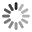The best jokes and joke writers!## Two Plus Two

A lawyer, an engineer and a mathematician were called in for a test. The engineer went in first and was asked, ''What is 2+2?'' The engineer thought awhile and finally answered, ''4.'' Then the mathematician was called in and was asked the same question. With little thought he replied, ''4.0.'' Then the lawyer was called in, and was asked the same question. The lawyer answered even quicker than the mathematician, ''What do you want it to be?''

## Irish Math

Q: In what town lives the mathematician who can only multiply by two?

A: Dublin.

## Prime Weed

Q: What do prime numbers and stoners have in common?

A: The higher they are, the more spaced out they get.

## Noah Multiply

After the flood, Noah tells the animals from the Arc to "go forth and multiply."

After a few months, Noah figures he better wander around and see how the animals are doing. Everybody is happy until he comes across a couple of snakes - they are quite downcast and not very happy. Noah asks what wrong, and they say "We are Adders, so we can't multiply!"

Noah rubs his chin for a few moments, and then goes into the forest, cuts down a couple of trees, and makes a table out of them.

Then he puts the snakes up on the platform he has made, and says "Now you should be happy. Everybody knows that adders can multiply with log tables!"

## Ball Size

A mathematician, a physicist, and an engineer are all given identical rubber balls and told to find the volume. They are given anything they want to measure it, and have all the time they need. The mathematician pulls out a measuring tape and records the circumference. He then divides by two times pi to get the radius, cubes that, multiplies by pi again, and then multiplies by four-thirds and thereby calculates the volume. The physicist gets a bucket of water, places 1.00000 gallons of water in the bucket, drops in the ball, and measures the displacement to six significant figures. And the engineer? He writes down the serial number of the ball, and looks it up.